# Heaviside step function

In mathematics, physics, and engineering the Heaviside step function is the following function,$H(x) = \begin{cases} 1 &\quad\hbox{if}\quad x > 0\\ 0 &\quad\hbox{if}\quad x < 0\\ \end{cases}$

The function is undetermined for x = 0, sometimes one defines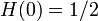$H(0) = 1/2\,$.

From the definition it follows immediately that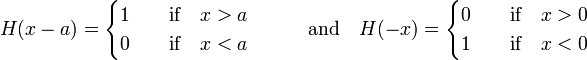$H(x-a) = \begin{cases} 1 &\quad\hbox{if}\quad x > a\\ 0 &\quad\hbox{if}\quad x < a\\ \end{cases} \qquad\quad\hbox{and}\quad H(-x) = \begin{cases} 0 &\quad\hbox{if}\quad x > 0\\ 1 &\quad\hbox{if}\quad x < 0\\ \end{cases}$

The function is named after the English mathematician Oliver Heaviside.

## Derivative

Note that a block ("boxcar") function BΔ of width Δ and height 1/Δ can be given in terms of step functions (for positive Δ), namely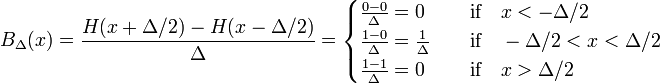$B_\Delta(x) = \frac{ H(x+\Delta/2) - H(x-\Delta/2)}{\Delta}= \begin{cases} \frac{0 - 0}{\Delta} = 0 & \quad\hbox{if}\quad x < -\Delta/2 \\ \frac{1 - 0}{\Delta} = \frac{1}{\Delta} & \quad\hbox{if}\quad -\Delta/2 < x < \Delta/2 \\ \frac{1 - 1}{\Delta} = 0 & \quad\hbox{if}\quad x > \Delta/2 \\ \end{cases}$

Knowing this, the derivative of H follows easily$\frac{dH(x)}{dx} = \lim_{\Delta\rightarrow 0} \frac{H(x+\Delta/2) -H(x-\Delta/2)}{\Delta} = \lim_{\Delta\rightarrow 0} B_\Delta(x) =\delta(x),$

where δ(x) is the Dirac delta function, which may be defined as the block function in the limit of zero width, see the article on the Dirac delta function.

The step function is a generalized function (a distribution). When H(x) is multiplied under the integral by the derivative of an arbitrary differentiable function f(x) that vanishes for plus/minus infinity, the result of the integral is minus the function value for x = 0,$\int_{-\infty}^{\infty} H(x) \frac{df(x)}{dx} \mathrm{d}x = - \int_{-\infty}^{\infty} \frac{dH(x)}{dx} f(x) \mathrm{d}x = - \int_{-\infty}^{\infty} \delta(x) f(x) \mathrm{d}x = -f(0).$

Here the "turnover rule" for d/dx is used, which may be proved by integration by parts and which holds when f(x) vanishes at the integration limits.

## H(x) as limit of arctan

In the figure it is shown that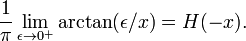$\frac{1}{\pi} \lim_{\epsilon \rightarrow 0^+} \arctan(\epsilon/x) = H(-x).$

Note here that the function arctan returns angles on the full interval 0 to 2π. In particular, if a point x + iy in the complex plane has x < 0 and y approaches zero from above, then the function arctan returns a value approaching π. Most computer languages use a two parameter function for this form of the inverse tangent.

## Fourier transform$\mathcal{F}(H) \equiv \sqrt{\frac{1}{2\pi}} \int_{-\infty}^{\infty} e^{-iux}H(x) dx = \sqrt{\frac{1}{2\pi}} \left( \pi \delta(u) - i \mathrm{P}\Big(\frac{1}{u}\Big)\right),$

where δ(u) is the Dirac delta function and P stands for the Cauchy principal value.

### Proof

Write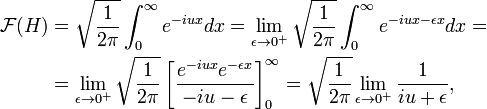\begin{align} \mathcal{F}(H) &= \sqrt{\frac{1}{2\pi}} \int_{0}^{\infty} e^{-iux} dx = \lim_{\epsilon \rightarrow 0^+} \sqrt{\frac{1}{2\pi}} \int_{0}^{\infty} e^{-iux-\epsilon x} dx = \\ &= \lim_{\epsilon \rightarrow 0^+} \sqrt{\frac{1}{2\pi}} \left[ \frac{e^{-iux}e^{-\epsilon x}}{-iu-\epsilon} \right]^{\infty}_0 =\sqrt{\frac{1}{2\pi}} \lim_{\epsilon \rightarrow 0^+} \frac{1}{iu+\epsilon}, \end{align}

where we used$\lim_{x \rightarrow \infty} e^{-iux}\,e^{-\epsilon x} = 0,\quad \epsilon > 0.$

Now use the following relation,$\lim_{\epsilon \rightarrow 0^+} \frac{-i}{u-i\epsilon} = -i \mathrm{P}\Big(\frac{1}{u}\Big) + \pi \delta(u)$

and the result is proved.

In order to prove the last relation we write a complex number in polar form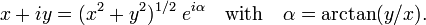$x+iy = (x^2+y^2)^{1/2}\; e^{i\alpha}\quad \hbox{with}\quad \alpha = \arctan(y/x).$

Take the natural logarithm and the limit for y → 0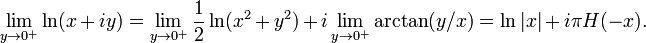$\lim_{y \rightarrow 0^+} \ln(x+iy) = \lim_{y \rightarrow 0^+} \frac{1}{2} \ln(x^2+y^2) + i\lim_{y \rightarrow 0^+} \arctan(y/x) = \ln|x| + i \pi H(-x).$.

Differentiation of the last expression gives gives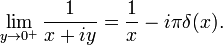$\lim_{y \rightarrow 0^+} \frac{1}{x+iy} = \frac{1}{x} -i\pi \delta(x).$

In fact, the functions in this expression are distributions (generalized functions) and are to be used in an integrand multiplied by a well-behaved function f(x). Since 1/x is singular for x = 0, we must take the Cauchy principal value of the integral, i.e., exclude the singularity from the integral; we make the replacement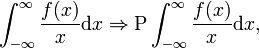$\int_{-\infty}^{\infty} \frac{f(x)}{x} \mathrm{d}x \Rightarrow \mathrm{P}\int_{-\infty}^{\infty} \frac{f(x)}{x} \mathrm{d}x,$

and the result follows.